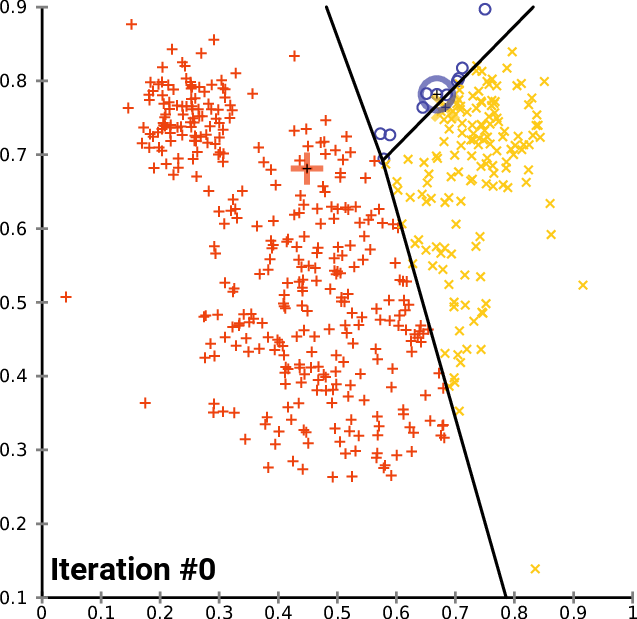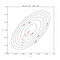# K-means Clustering

From scratch explanation and implementation

K-means Clustering is an unsupervised machine learning technique. It aims to partition n observations into k clusters.

Kmeans algorithm is an iterative algorithm that tries to partition the dataset into K distinct non-overlapping subgroups (clusters) where each data point belongs to only one groupSource : Wikipedia

GOAL → Partition data among k number of clusters, where k is known to us.

# Procedure of kmeans Algorithm

1. Randomly initialize K centres (centroids) .
2. Iterating over the dataset till we reach maximum number of iterations or before if there is change in centroids of clusters.
3. Calculating Euclidian distance of each point and from every cluster centre. Assign each point to a cluster whosoever distance is minimum. This step is called E-step (Expectation step). {K-Means is a special case of Expectation Algorithm}
4. Compute the mean of every cluster and then update it with centroid of that cluster. This step is called M-step(Maximization step)
5. Repeat these till we reach maximum iteration or to our correct centroid.

K-means can be stated as E-M (Expectation-Maximization) algorithm. E-step populates points while M-step updates centre.

## Maths Behind K-means

We introduce wik here as we are going to add distance for a cluster that point belongs not other clusters.

We first minimize J with respect to wik keeping μk fixed. Then we minimize J w.r.t. μk and treat wik fixed.

When we are differentiating J w.r.t. wik first and update assignments of cluster which is E-step. Then we differentiate J w.r.t. μk and update the centroids which is called M-step.

E-step is

M-step is

This is same as us taking mean as denominator says the summation of 1’s and 0’s that is number of points in kth cluster. while numerator is sum of values of those points in that cluster.

This method of minimizing error and finding wik and μk is called Coordinate Descent which has a graph like thisSource : Wikipedia

CODE for K-means

`# Distance between two points Euclidian Distancedef distance(a1,a2):    return np.sqrt(np.sum((a1-a2)**2))class KMeans():    def __init__(self,k=5,max_iter=100):        self.k= k        self.max_iter=max_iter        self.clusters = {}        self.label = []            def initialization(self,X):        for i in range(self.k):            center=np.zeros((2,))            cx = np.random.uniform(low=38.8250, high=39.0000)            cy = np.random.uniform(low=-76.9, high=-77.15)            center = cx            center = cy            #center = 10*(2*np.random.random((X.shape,))-1)            points = []            cluster = {                'center':center,                'points':points,                'id'    :i            }            self.clusters[i]=cluster        self.label = np.zeros((X.shape,1))        def assignPointTOClusters(self,X):        for i in range(X.shape):            dist = []            curr_x = X[i]                    for ki in range(self.k):                d = distance(curr_x,self.clusters[ki]['center'])                dist.append(d)                        current_cluster = np.argmin(dist)            self.clusters[current_cluster]['points'].append(curr_x)            self.label[i]=(self.clusters[current_cluster]['id'])                def check(self,old_c,new_c):        distances = [distance(old_c[i], new_c[i]) for i in range(self.k)]        return sum(distances) == 0            def updateClusters(self):        for kx in range(self.k):            pts = np.array(self.clusters[kx]['points'])                        if pts.shape>0: # If cluster has some nonzero points                new_u = pts.mean(axis=0)                self.clusters[kx]['center'] = new_u                # Clear the list                self.clusters[kx]['points'] = []        def plotClusters(self):        for kx in range(self.k):            print(len(self.clusters[kx]['points']))            pts = np.array(self.clusters[kx]['points'])            # plot points , cluster center            try:                plt.scatter(pts[:,0],pts[:,1])            except:                pass            uk = self.clusters[kx]['center']            plt.scatter(uk,uk,color='black',marker="*")        plt.show()                def fit(self,X):        print(self.k)        self.initialization(X)        for i in range(self.max_iter):            print("i is ",i)            self.assignPointTOClusters(X)            self.plotClusters()            old_c = [self.clusters[i]['center'] for i in range(self.k)]            self.updateClusters()            new_c = [self.clusters[i]['center'] for i in range(self.k)]            if self.check(old_c,new_c):                break`

# K-Means++ : Just a Introduction

• To overcome this problem, we use technique called K-Means++ (described in paper Robust Seed Selection for K-Means type of Algorithms) which chooses initial centers so that they are statiscallly close to final ones.

## Analytics Vidhya

Analytics Vidhya is a community of Analytics and Data…

## Sign up for Analytics Vidhya News Bytes

### By Analytics Vidhya

Latest news from Analytics Vidhya on our Hackathons and some of our best articles! Take a look.

By signing up, you will create a Medium account if you don’t already have one. Review our Privacy Policy for more information about our privacy practices.

Check your inbox
Medium sent you an email at to complete your subscription.

## Analytics Vidhya

Analytics Vidhya is a community of Analytics and Data Science professionals. We are building the next-gen data science ecosystem https://www.analyticsvidhya.com

Written by

## Aditri Srivastava

You are more powerful than you know## Analytics Vidhya

Analytics Vidhya is a community of Analytics and Data Science professionals. We are building the next-gen data science ecosystem https://www.analyticsvidhya.com

## More From Medium

Medium is an open platform where 170 million readers come to find insightful and dynamic thinking. Here, expert and undiscovered voices alike dive into the heart of any topic and bring new ideas to the surface. Learn more

Follow the writers, publications, and topics that matter to you, and you’ll see them on your homepage and in your inbox. Explore

If you have a story to tell, knowledge to share, or a perspective to offer — welcome home. It’s easy and free to post your thinking on any topic. Write on Medium